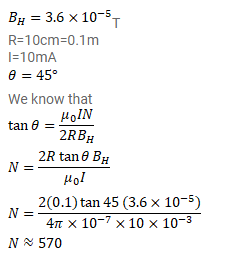# A tangent galvanometer shows a deflection of

Question:

A tangent galvanometer shows a deflection of $45^{\circ}$ when $10 \mathrm{~mA}$ of current is passed through it. If the horizontal component of the earth's magnetic field is $B_{H}=3.6 \times 10^{-5} \mathrm{~T}$ and radius of the coil is $10 \mathrm{~cm}$, find the number of turns in the coil.

Solution: